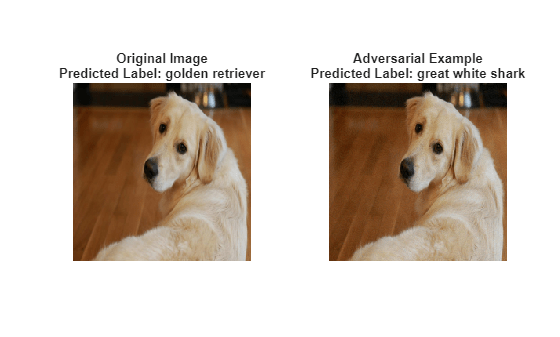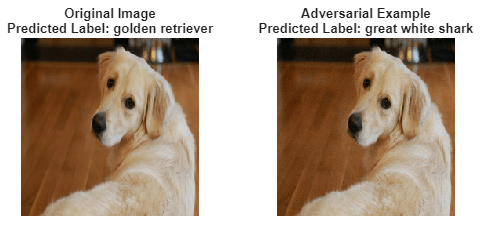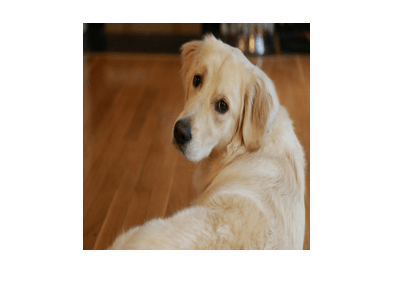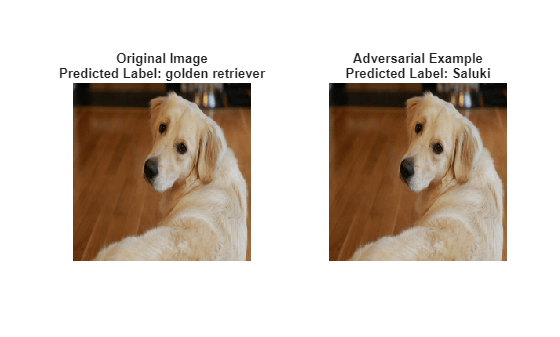Generate Untargeted and Targeted Adversarial Examples for Image Classification

This example shows how to use the fast gradient sign method (FGSM) and the basic iterative method (BIM) to generate adversarial examples for a pretrained neural network.

Neural networks can be susceptible to a phenomenon known as adversarial examples , where very small changes to an input can cause the input to be misclassified. These changes are often imperceptible to humans.

In this example, you create two types of adversarial examples:

• Untargeted — Modify an image so that it is misclassified as any incorrect class.

• Targeted — Modify an image so that it is misclassified as a specific class.

Load a network that has been trained on the ImageNet  data set and convert it to a dlnetwork.

net =squeezenet;

lgraph = layerGraph(net);
lgraph = removeLayers(lgraph,lgraph.Layers(end).Name);
dlnet = dlnetwork(lgraph);

Extract the class labels.

classes = categories(net.Layers(end).Classes);

Load an image to use to generate an adversarial example. The image is a picture of a golden retriever.

T = "golden retriever";

Resize the image to match the input size of the network.

inputSize = dlnet.Layers(1).InputSize;
img = imresize(img,inputSize(1:2));

figure
imshow(img)
title("Ground Truth: " + T)Prepare the image by converting it to a dlarray.

X = dlarray(single(img),"SSCB");

Prepare the label by one-hot encoding it.

T = onehotencode(T,1,'ClassNames',classes);
T = dlarray(single(T),"CB");

Create an adversarial example using the untargeted FGSM . This method calculates the gradient ${\nabla }_{X}L\left(X,T\right)$ of the loss function $L$, with respect to the image $X$ you want to find an adversarial example for, and the class label $T$. This gradient describes the direction to "push" the image in to increase the chance it is misclassified. You can then add or subtract a small error from each pixel to increase the likelihood the image is misclassified.

The adversarial example is calculated as follows:

${\mathit{X}}_{\mathrm{adv}}=\mathit{X}+ϵ.\mathrm{sign}\left({\nabla }_{\mathit{X}}\mathit{L}\left(\mathit{X},\mathit{T}\right)\right)$.

Parameter $ϵ$ controls the size of the push. A larger $ϵ$ value increases the chance of generating a misclassified image, but makes the change in the image more visible. This method is untargeted, as the aim is to get the image misclassified, regardless of which class.

Calculate the gradient of the image with respect to the golden retriever class.

Set epsilon to 1 and generate the adversarial example.

epsilon = 1;

Predict the class of the original image and the adversarial image.

YPred = predict(dlnet,X);
YPred = onehotdecode(squeeze(YPred),classes,1)
YPred = categorical
golden retriever

Display the original image, the perturbation added to the image, and the adversarial image. If the epsilon value is large enough, the adversarial image has a different class label from the original image.The network correctly classifies the unaltered image as a golden retriever. However, because of perturbation, the network misclassifies the adversarial image as a labrador retriever. Once added to the image, the perturbation is imperceptible, demonstrating how adversarial examples can exploit robustness issues within a network.

A simple improvement to FGSM is to perform multiple iterations. This approach is known as the basic iterative method (BIM)  or projected gradient descent . For the BIM, the size of the perturbation is controlled by parameter $\alpha$ representing the step size in each iteration. This is as the BIM usually takes many, smaller, FGSM steps in the direction of the gradient. After each iteration, clip the perturbation to ensure the magnitude does not exceed $ϵ$. This method can yield adversarial examples with less distortion than FGSM.

When you use untargeted FGSM, the predicted label of the adversarial example can be very similar to the label of the original image. For example, a dog might be misclassified as a different kind of dog. However, you can easily modify these methods to misclassify an image as a specific class. Instead of maximizing the cross-entropy loss, you can minimize the mean squared error between the output of the network and the desired target output.

Generate a targeted adversarial example using the BIM and the great white shark target class.

targetClass = "great white shark";
targetClass = onehotencode(targetClass,1,'ClassNames',classes);

Increase the epsilon value to 5, set the step size alpha to 0.2, and perform 25 iterations. Note that you may have to adjust these settings for other networks.

epsilon = 5;
alpha = 0.2;
numIterations = 25;

Keep track of the perturbation and clip any values that exceed epsilon.

delta = zeros(size(X),'like',X);
for i = 1:numIterations

delta(delta > epsilon) = epsilon;
delta(delta < -epsilon) = -epsilon;
end

Predict the class of the targeted adversarial example.

great white shark

Display the original image, the perturbation added to the image, and the targeted adversarial image.Because of imperceptible perturbation, the network classifies the adversarial image as a great white shark.

To make the network more robust against adversarial examples, you can use adversarial training. For an example showing how to train a network robust to adversarial examples, see Train Image Classification Network Robust to Adversarial Examples.

Supporting Functions

Y = predict(dlnet,X);
Y = stripdims(squeeze(Y));
loss = crossentropy(Y,target,'DataFormat','CB');

end

Y = predict(dlnet,X);
Y = stripdims(squeeze(Y));
loss = mse(Y,target,'DataFormat','CB');

end

Show an image, the corresponding adversarial image, and the difference between the two (perturbation).

figure
subplot(1,3,1)
imgTrue = uint8(extractdata(image));
imshow(imgTrue)
title("Original Image" + newline + "Class: " + string(label))

subplot(1,3,2)
imshow(perturbation)
title("Perturbation")

subplot(1,3,3)
title("Adversarial Image (Epsilon = " + string(epsilon) + ")" + newline + ...
end

References

 Goodfellow, Ian J., Jonathon Shlens, and Christian Szegedy. “Explaining and Harnessing Adversarial Examples.” Preprint, submitted March 20, 2015. https://arxiv.org/abs/1412.6572.

 ImageNet. http://www.image-net.org.

 Szegedy, Christian, Wojciech Zaremba, Ilya Sutskever, Joan Bruna, Dumitru Erhan, Ian Goodfellow, and Rob Fergus. “Intriguing Properties of Neural Networks.” Preprint, submitted February 19, 2014. https://arxiv.org/abs/1312.6199.

 Kurakin, Alexey, Ian Goodfellow, and Samy Bengio. “Adversarial Examples in the Physical World.” Preprint, submitted February 10, 2017. https://arxiv.org/abs/1607.02533.

 Madry, Aleksander, Aleksandar Makelov, Ludwig Schmidt, Dimitris Tsipras, and Adrian Vladu. “Towards Deep Learning Models Resistant to Adversarial Attacks.” Preprint, submitted September 4, 2019. https://arxiv.org/abs/1706.06083.